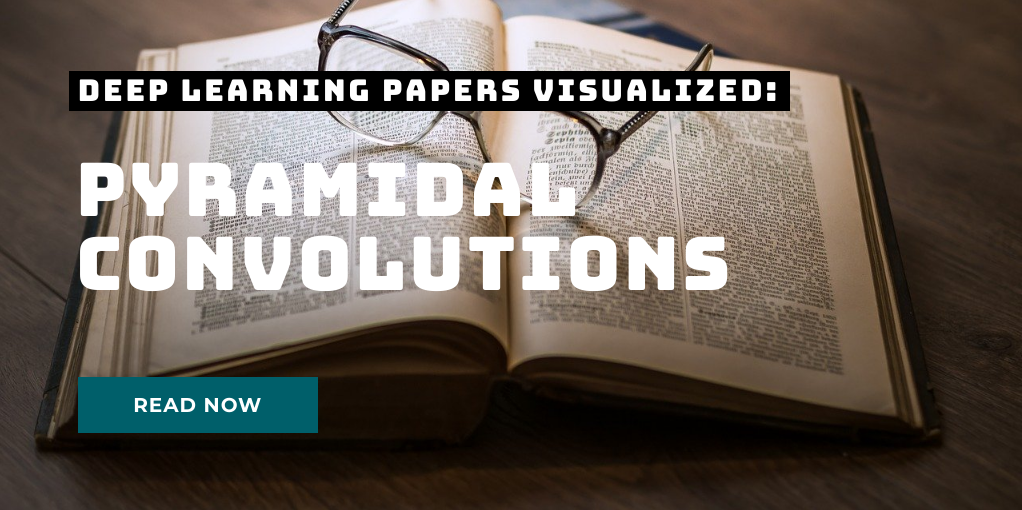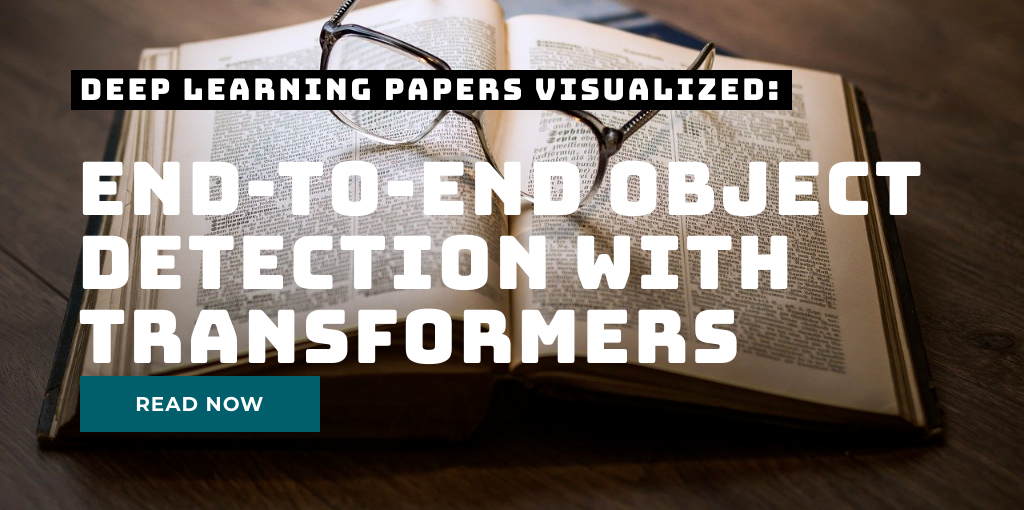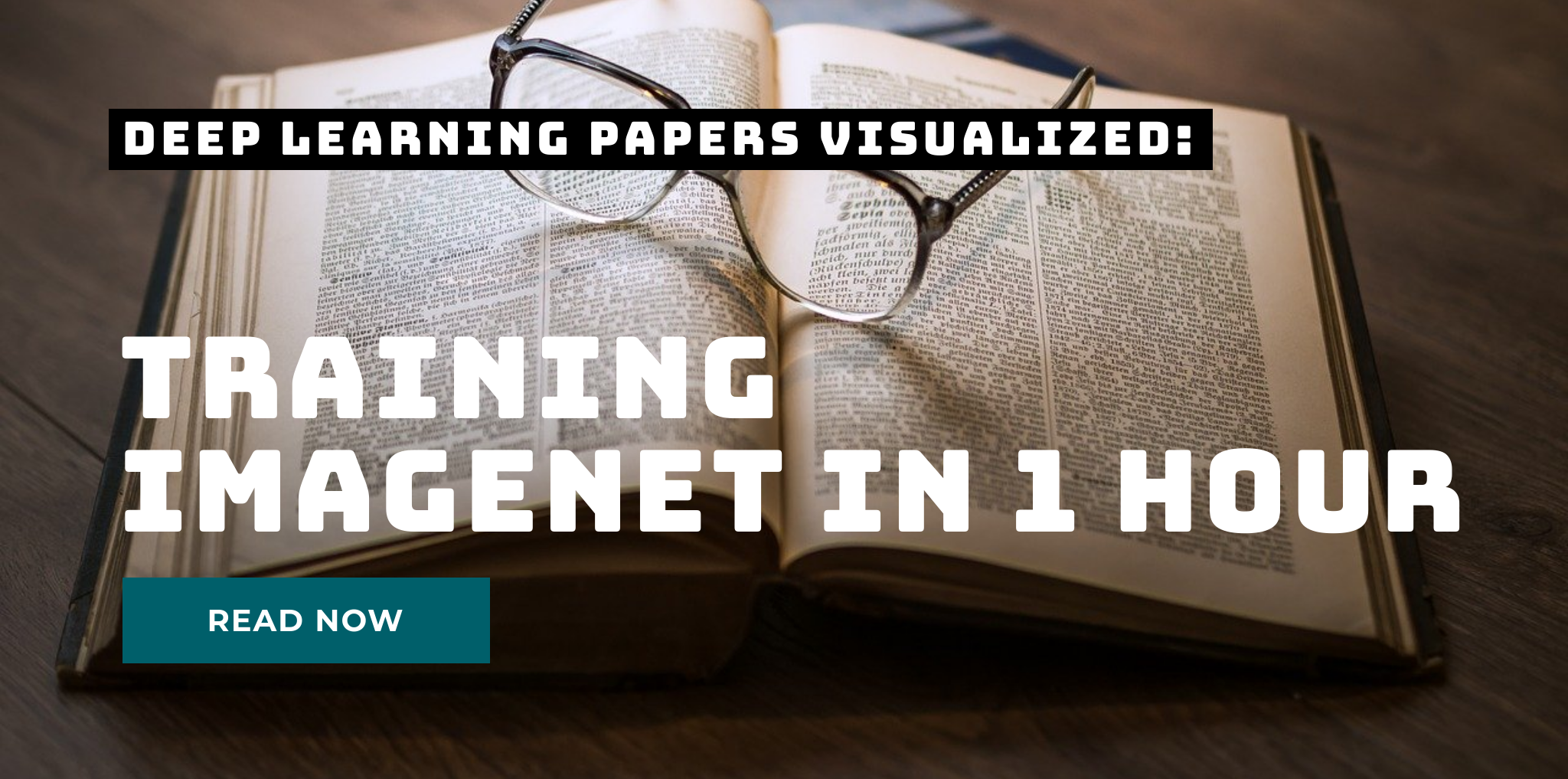# Depthwise Separable Convolutions in PyTorch

In many neural network architectures like MobileNets, depthwise separable convolutions are used instead of regular convolutions. They have been shown to yield similar performance while being much more efficient in terms of using much less parameters and less floating point operations (FLOPs). Today, we will take a look at the difference of depthwise separable convolutions to standard convolutions and will analyze where the efficiency comes from. Short recap: standard convolution In standard convolutions, we are analyzing an input map of height H and width W comprised of C channels.

# Pyramidal Convolution: Rethinking Convolutional Neural Networks for Visual RecognitionToday’s paper: Pyramidal Convolution by Duta et al. This is the third paper of the new series Deep Learning Papers visualized and it’s about using convolutions in a pyramidal style to capture information of different magnifications from an image. The authors show how a pyramidal convolution can be constructed and apply it to several problems in the visual domain. What’s really interesting is that the number of parameters can be kept the same while performance tends to improve.

# End-to-End object detection with transformersToday’s paper: End-to-End object detection with transformers by Carion et al. This is the second paper of the new series Deep Learning Papers visualized and it’s about using a transformer approach (the current state of the art in the domain of speech) to the domain of vision. More specifically, the paper is concerned with object detection and here is the link to the paper of Carion et al. on arxiv.

# Accurate, Large Minibatch SGD: Training ImageNet in 1 HourNew blog series: Deep Learning Papers visualized This is the first post of a new series I am starting where I explain the content of a paper in a visual picture-based way. To me, this helps tremendously to better grasp the ideas and remember them and I hope this will be the same for many of you as well. Today’s paper: Accurate, Large Minibatch SGD: Training ImageNet in 1 Hour by Goyal et al.

# Eigenvectors and eigenvalues in machine learning

As a data scientist, you are dealing a lot with linear algebra and in particular the multiplication of matrices. Important properties of a matrix are its eigenvalues and corresponding eigenvectors. So let’s explore those a bit to get a better intuition of what they tell you about the transformation. We will just need numpy and a plotting library and create a set of points that make up a rectangle (5 points, so they are visually connected in the plot):